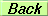# SEMINAR

The remarkable role of the vector-like quark doublet in the Cabibbo angle and W-mass anomalies

Benedetta Belfatto

(SISSA, Trieste)

Room 2.8.3, IST, Physics Dept.
Tuesday, June 27th, 2023 at 04:00 PM

Abstract

The combination of precise determinations of $V_{us}$ and $V_{ud}$ hints towards a violation of the CKM first row unitarity at about $3\sigma$ level. A possible explanation can be the mixing of SM quarks with vector-like quarks, up or down type $SU(2)_L$ singlets or $SU(2)_L$ doublet, with mass of few TeV, having large enough mixings with the lighter quarks. A different solution may come from the introduction of the gauge horizontal family symmetry acting between the lepton families and spontaneously broken at the scale of about 6 TeV. Another tension in the value of the Cabibbo angle is also reported at $3\sigma$ level, between two determinations of $V_{us}$ obtained from semi-leptonic and leptonic kaon decays. A vector-like doublet can account for this discrepancy. Although one extra multiplet cannot entirely explain all the Cabibbo anomalies, a combination such as two species of isodoublets, or one isodoublet and up or/and down type isosinglet can be a complete solution.

Moreover, the recent measurement of the $W$-boson mass by the CDF Collaboration exhibits significant tension with the SM prediction, intensifying the conflict that may arise in models addressing the CKM unitarity violation. One vector-like doublet of quarks mixing with light quarks and the top quark can be the common origin of the CKM unitarity deficit or the tension in $V_{us}$ determinations and the positive shift in $W$-boson mass, without conflicting with flavour-changing phenomena and electroweak observables.

(Seminar slides)With support from FCT through project UIDB/00777/2020